# 12.3 The parabola  (Page 7/11)

 Page 7 / 11

${\left(y+4\right)}^{2}=16\left(x+4\right)$

${y}^{2}+12x-6y+21=0$

${\left(y-3\right)}^{2}=-12\left(x+1\right),V:\left(-1,3\right);F:\left(-4,3\right);d:x=2$

${x}^{2}-4x-24y+28=0$

$5{x}^{2}-50x-4y+113=0$

${\left(x-5\right)}^{2}=\frac{4}{5}\left(y+3\right),V:\left(5,-3\right);F:\left(5,-\frac{14}{5}\right);d:y=-\frac{16}{5}$

${y}^{2}-24x+4y-68=0$

${x}^{2}-4x+2y-6=0$

${\left(x-2\right)}^{2}=-2\left(y-5\right),V:\left(2,5\right);F:\left(2,\frac{9}{2}\right);d:y=\frac{11}{2}$

${y}^{2}-6y+12x-3=0$

$3{y}^{2}-4x-6y+23=0$

${\left(y-1\right)}^{2}=\frac{4}{3}\left(x-5\right),V:\left(5,1\right);F:\left(\frac{16}{3},1\right);d:x=\frac{14}{3}$

${x}^{2}+4x+8y-4=0$

## Graphical

For the following exercises, graph the parabola, labeling the focus and the directrix.

$x=\frac{1}{8}{y}^{2}$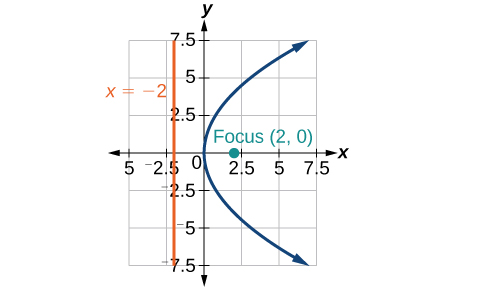$y=36{x}^{2}$

$y=\frac{1}{36}{x}^{2}$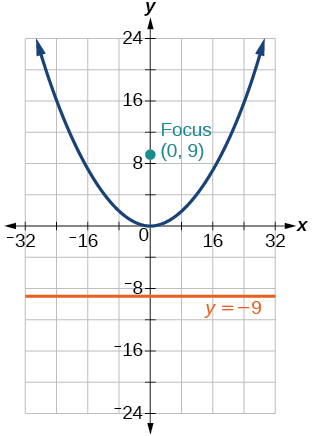$y=-9{x}^{2}$

${\left(y-2\right)}^{2}=-\frac{4}{3}\left(x+2\right)$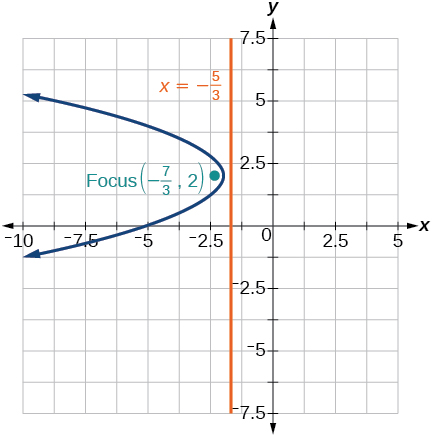$-5{\left(x+5\right)}^{2}=4\left(y+5\right)$

$-6{\left(y+5\right)}^{2}=4\left(x-4\right)$${y}^{2}-6y-8x+1=0$

${x}^{2}+8x+4y+20=0$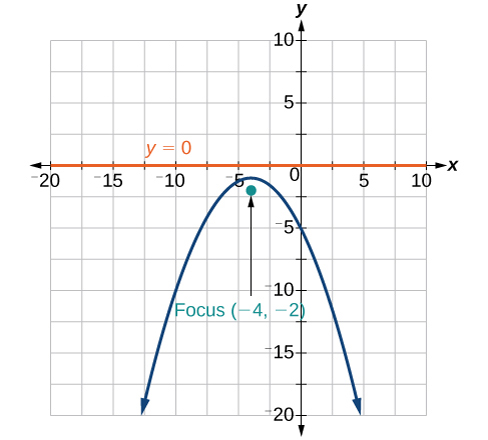$3{x}^{2}+30x-4y+95=0$

${y}^{2}-8x+10y+9=0$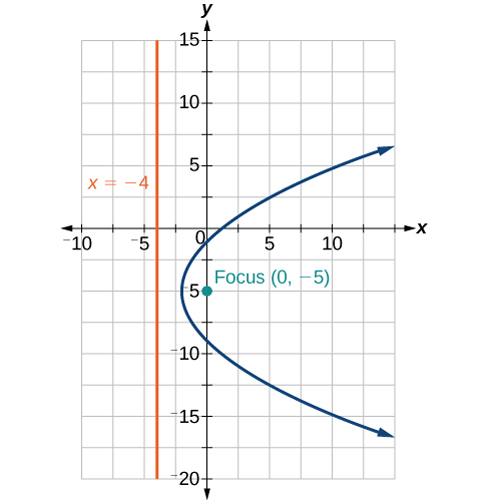${x}^{2}+4x+2y+2=0$

${y}^{2}+2y-12x+61=0$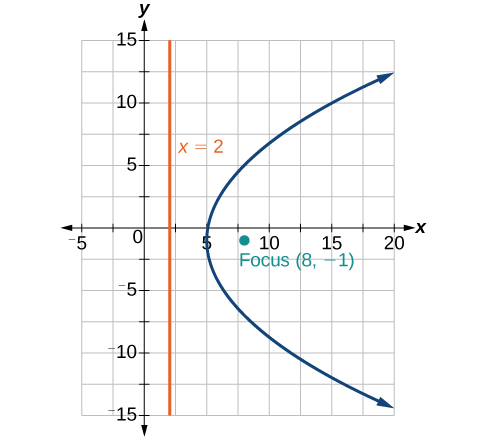$-2{x}^{2}+8x-4y-24=0$

For the following exercises, find the equation of the parabola given information about its graph.

Vertex is $\text{\hspace{0.17em}}\left(0,0\right);$ directrix is $\text{\hspace{0.17em}}y=4,$ focus is $\text{\hspace{0.17em}}\left(0,-4\right).$

${x}^{2}=-16y$

Vertex is $\text{\hspace{0.17em}}\left(0,0\right);\text{\hspace{0.17em}}$ directrix is $\text{\hspace{0.17em}}x=4,$ focus is $\text{\hspace{0.17em}}\left(-4,0\right).$

Vertex is $\text{\hspace{0.17em}}\left(2,2\right);\text{\hspace{0.17em}}$ directrix is $\text{\hspace{0.17em}}x=2-\sqrt{2},$ focus is $\text{\hspace{0.17em}}\left(2+\sqrt{2},2\right).$

${\left(y-2\right)}^{2}=4\sqrt{2}\left(x-2\right)$

Vertex is $\text{\hspace{0.17em}}\left(-2,3\right);\text{\hspace{0.17em}}$ directrix is $\text{\hspace{0.17em}}x=-\frac{7}{2},$ focus is $\text{\hspace{0.17em}}\left(-\frac{1}{2},3\right).$

Vertex is $\text{\hspace{0.17em}}\left(\sqrt{2},-\sqrt{3}\right);$ directrix is $\text{\hspace{0.17em}}x=2\sqrt{2},$ focus is $\text{\hspace{0.17em}}\left(0,-\sqrt{3}\right).$

${\left(y+\sqrt{3}\right)}^{2}=-4\sqrt{2}\left(x-\sqrt{2}\right)$

Vertex is $\text{\hspace{0.17em}}\left(1,2\right);\text{\hspace{0.17em}}$ directrix is $\text{\hspace{0.17em}}y=\frac{11}{3},$ focus is $\text{\hspace{0.17em}}\left(1,\frac{1}{3}\right).$

For the following exercises, determine the equation for the parabola from its graph.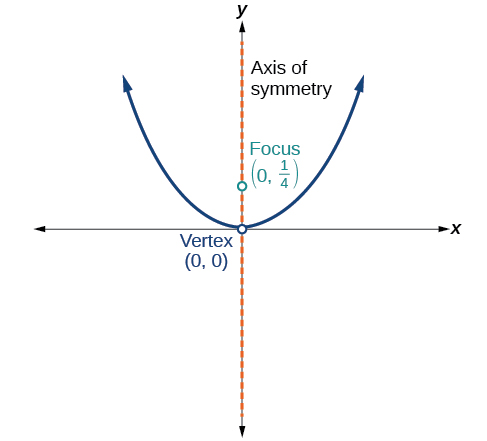${x}^{2}=y$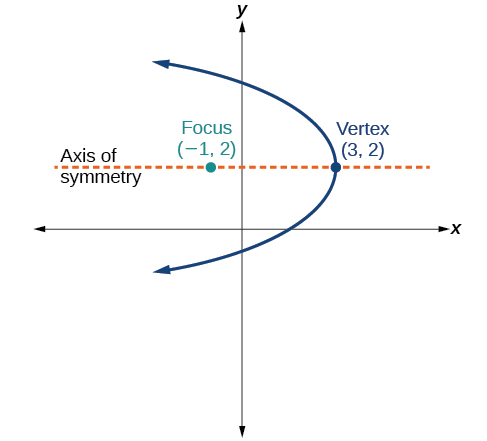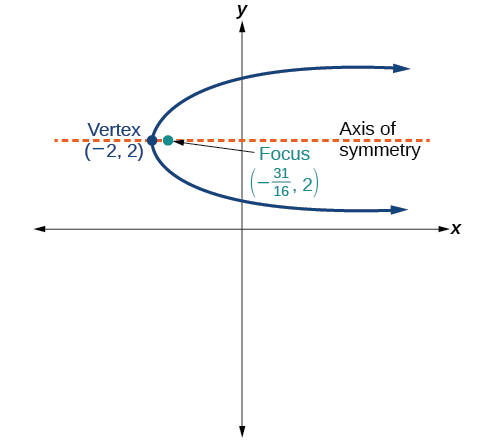${\left(y-2\right)}^{2}=\frac{1}{4}\left(x+2\right)$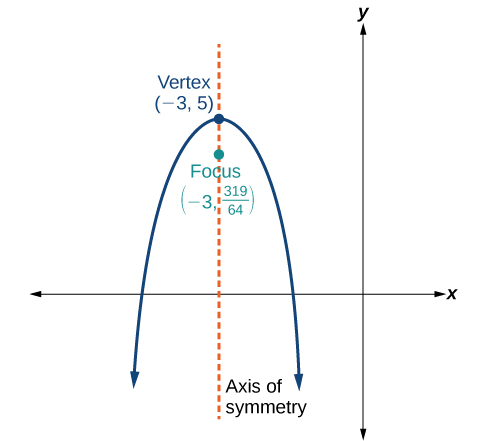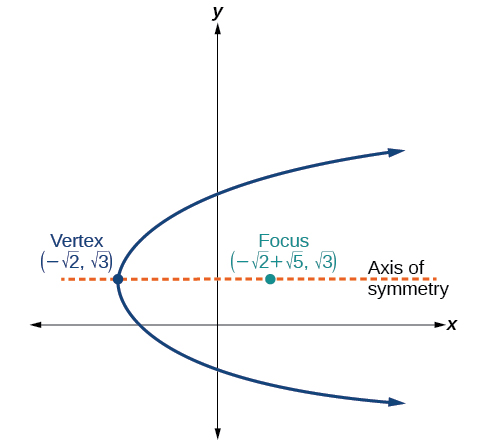${\left(y-\sqrt{3}\right)}^{2}=4\sqrt{5}\left(x+\sqrt{2}\right)$

## Extensions

For the following exercises, the vertex and endpoints of the latus rectum of a parabola are given. Find the equation.

${y}^{2}=-8x$

${\left(y+1\right)}^{2}=12\left(x+3\right)$

## Real-world applications

The mirror in an automobile headlight has a parabolic cross-section with the light bulb at the focus. On a schematic, the equation of the parabola is given as $\text{\hspace{0.17em}}{x}^{2}=4y.\text{\hspace{0.17em}}$ At what coordinates should you place the light bulb?

$\left(0,1\right)$

If we want to construct the mirror from the previous exercise such that the focus is located at $\text{\hspace{0.17em}}\left(0,0.25\right),$ what should the equation of the parabola be?

A satellite dish is shaped like a paraboloid of revolution. This means that it can be formed by rotating a parabola around its axis of symmetry. The receiver is to be located at the focus. If the dish is 12 feet across at its opening and 4 feet deep at its center, where should the receiver be placed?

At the point 2.25 feet above the vertex.

Consider the satellite dish from the previous exercise. If the dish is 8 feet across at the opening and 2 feet deep, where should we place the receiver?

A searchlight is shaped like a paraboloid of revolution. A light source is located 1 foot from the base along the axis of symmetry. If the opening of the searchlight is 3 feet across, find the depth.

0.5625 feet

If the searchlight from the previous exercise has the light source located 6 inches from the base along the axis of symmetry and the opening is 4 feet, find the depth.

An arch is in the shape of a parabola. It has a span of 100 feet and a maximum height of 20 feet. Find the equation of the parabola, and determine the height of the arch 40 feet from the center.

${x}^{2}=-125\left(y-20\right),$ height is 7.2 feet

If the arch from the previous exercise has a span of 160 feet and a maximum height of 40 feet, find the equation of the parabola, and determine the distance from the center at which the height is 20 feet.

An object is projected so as to follow a parabolic path given by $\text{\hspace{0.17em}}y=-{x}^{2}+96x,$ where $\text{\hspace{0.17em}}x\text{\hspace{0.17em}}$ is the horizontal distance traveled in feet and $\text{\hspace{0.17em}}y\text{\hspace{0.17em}}$ is the height. Determine the maximum height the object reaches.

2304 feet

For the object from the previous exercise, assume the path followed is given by $\text{\hspace{0.17em}}y=-0.5{x}^{2}+80x.\text{\hspace{0.17em}}$ Determine how far along the horizontal the object traveled to reach maximum height.

#### Questions & Answers

The sequence is {1,-1,1-1.....} has
amit Reply
circular region of radious
Kainat Reply
how can we solve this problem
Joel Reply
Sin(A+B) = sinBcosA+cosBsinA
Eseka Reply
Prove it
Eseka
Please prove it
Eseka
hi
Joel
June needs 45 gallons of punch. 2 different coolers. Bigger cooler is 5 times as large as smaller cooler. How many gallons in each cooler?
Arleathia Reply
find the sum of 28th term of the AP 3+10+17+---------
Prince Reply
I think you should say "28 terms" instead of "28th term"
Vedant
if sequence sn is a such that sn>0 for all n and lim sn=0than prove that lim (s1 s2............ sn) ke hole power n =n
SANDESH Reply
write down the polynomial function with root 1/3,2,-3 with solution
Gift Reply
if A and B are subspaces of V prove that (A+B)/B=A/(A-B)
Pream Reply
write down the value of each of the following in surd form a)cos(-65°) b)sin(-180°)c)tan(225°)d)tan(135°)
Oroke Reply
Prove that (sinA/1-cosA - 1-cosA/sinA) (cosA/1-sinA - 1-sinA/cosA) = 4
kiruba Reply
what is the answer to dividing negative index
Morosi Reply
In a triangle ABC prove that. (b+c)cosA+(c+a)cosB+(a+b)cisC=a+b+c.
Shivam Reply
give me the waec 2019 questions
Aaron Reply
the polar co-ordinate of the point (-1, -1)
Sumit Reply

### Read also:

#### Get the best Algebra and trigonometry course in your pocket!

Source:  OpenStax, Algebra and trigonometry. OpenStax CNX. Nov 14, 2016 Download for free at https://legacy.cnx.org/content/col11758/1.6
Google Play and the Google Play logo are trademarks of Google Inc.

Notification Switch

Would you like to follow the 'Algebra and trigonometry' conversation and receive update notifications?ByByBy